Atoms

"All the world is made of atoms"

Introduction

In 1803 John Dalton used the ideas of matter being made of indivisible components(atoms from Greek atomos indivisible) to describe why chemical elements (elements are indivisible by chemistry according to Lavoisier) always combine in small whole integer ratios.

For example why two volumes of hydrogen gas plus one volume of oxygen gas create two volumes of water gas. (check it!)

Atomic theory went from Dalton's model which gave great structure to chemistry but which most scientists believed was just an untestable model to a much stronger model in several steps. First step in strengthening the existence of atoms was the observation in 1827 by Robert Brown of brownian motion. In 1905 Einstein figured out how to analyze brownian motion to obtain the size of atoms. After Einstein Jean Perrin did the work to compute the size of atoms.

From Brownian motion through Einstein to Atoms: http://www.youtube.com/watch?v=Vje3CsKWVNc

Ernest Rutherford probed atoms of gold with alpha particles (helium nuclei) and discovered the nucleus of atoms in 1911.

Video of a model of Rutherford's atom and nucleus http://www.youtube.com/watch?v=wzALbzTdnc8

Bohr created a model of how electrons orbit the nucleus in 1913 that predicted the energies of the spectral lines of hydrogen.

Today we can image individual atoms.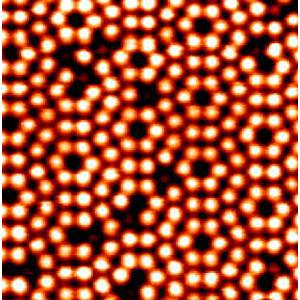Silicon atoms on a surface via scanning tunneling microscopy, STM.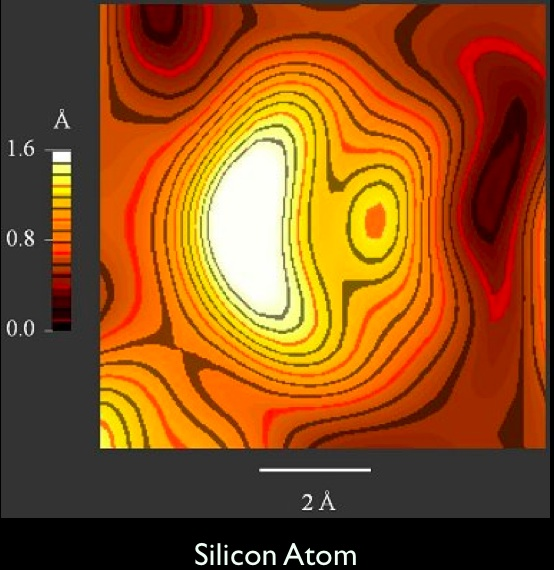An STM topo map of a single silicon atom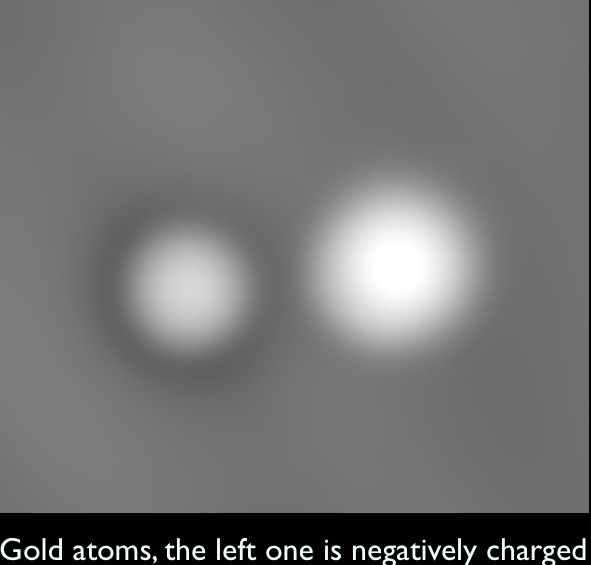An image of a gold atom and ion via scanning electron microscopy.

An atom is made from a nucleus containing protons and neutrons surrounded by electrons. (Hydrogen atoms have no neutrons)

For 300,000 years after the big bang there were no atoms. Just hydrogen and helium nuclei and separate electrons. After 300,000 years the universe cooled to the temperature where electrons could join nuclei to make atoms, and the universe has been dominated by atoms ever since.

The protons are positively charged and the electrons are negatively charged, they have exactly equal and opposite charges so that an atom with equal numbers of positive and negative charges is neutral or uncharged.

All neutral atoms are about 0.1 nanometers in diameter, r = 10^-10 m ( or 1 angstrom, a unit that the System International tried to remove but which is still hanging on until the last of the spectroscopists die.)

The nucleus is on the order of 1 femtometer, 1 fm, in radius informally called a fermi. (Hydrogen is 1 fermi in radius other atomic nuclei grow larger in proportion to the cube root of the sum of the number of protons and neutrons heavy nuclei may be 10 fm in radius.). The nucleus is 100,000 times smaller in radius than the atom, and 10^-15 times the volume of the atom, yet it contains more than 99.9%  of the mass of the atom.

What is the relationship between the size of the nucleus and the atom? If the nucleus were the size of the head of a pin, 1mm in diameter the atom would be 100m in diameter, about the length of a football field.

Q1. Why doesn't the electron fall into the nucleus since electrons and nuclei are oppositely charged, and opposite charges attract?

The electron does fall into the nucleus, it just always misses. Just as the moon is attracted by the earth and always falls towards the earth yet always misses. We call this an orbit.

Q2 But wait, whenever a charge accelerates, as the electron would in a circular orbit, it emits electromagnetic radiation which carries away energy from the orbit why doesn't the electron spiral into the nucleus?(Aside, binary neutron stars do radiate gravity waves and they do spiral together.)

Bohr's answer. In free space electrons are probability density waves, sinewaves of probability. These electrons have a deBroglie wavelength that depends on their momentum. Higher momentum  electrons have a shorter wavelength.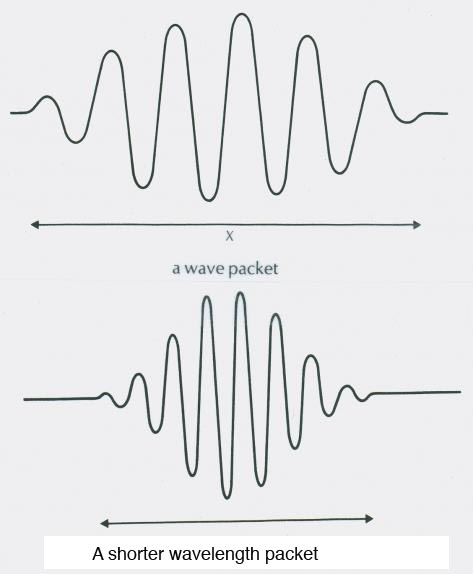Drawings of the probability wave of an electron in a vacuum. The square of this function gives the probability per unit volume of finding the electron at that position in space.

An image of a photon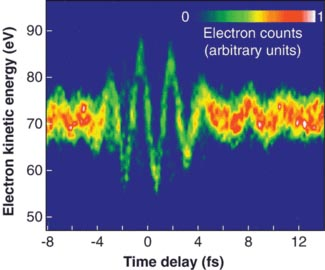An image of a photon wavepacket taken using electrons. fs = femtoseconds.

What are these waves in?

In 1900 they were waves in electric fields. The image above shows an image taken using the interaction of electric fields and electrons.

By 1930 they were waves in probability density.

The square of the wavefunction gives the probability per unit volume of finding the particle at that location.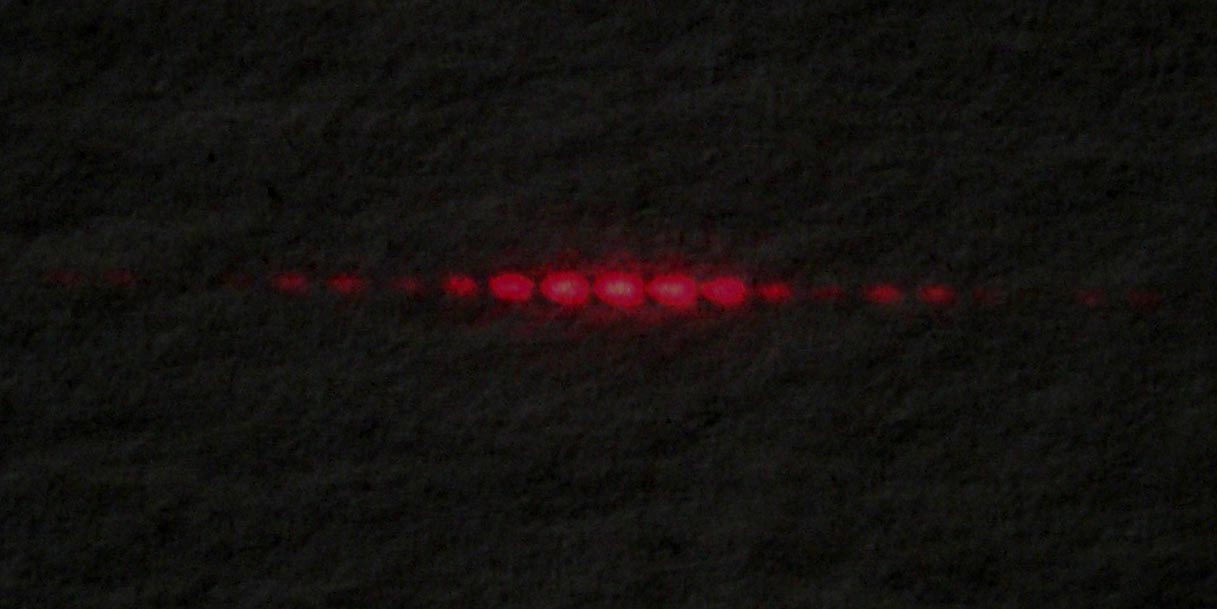The two slit diffraction pattern shows that photon arrival on a screen is controlled by the probability density of the photon waves.

Wrap one of these sinewaves around an atomic nucleus in an orbit if it exactly fits it makes a standing wave, this standing wave does not radiate electromagnetic waves and an electron can remain in such an orbit forever. Bohr stated that the electrons had to orbit with specific quanta of angular momentum.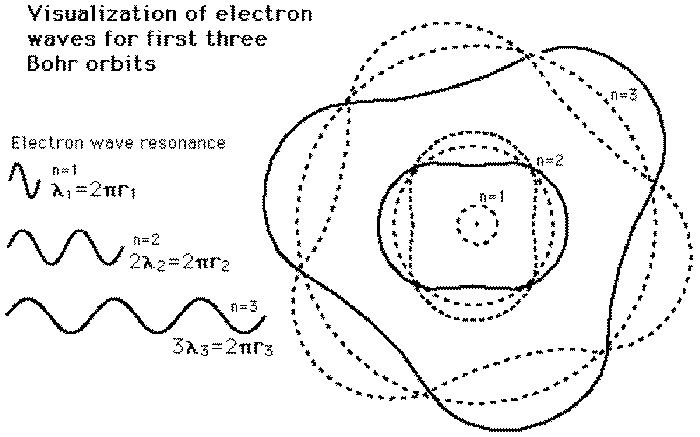As an exercise draw the n = 1 Bohr orbital yourself.

Bohr model applet http://www.walter-fendt.de/ph11e/bohrh.htm

Why are they not equally spaced. use a gravity well, roll balls or coins around the well. Gravity and electric forces are both inverse square and so produce the same orbits obeying Kepler's laws. Outer orbits require slower velocity and so longer deBroglie wavelengths. Thus they are much larger in radius, for example the n=2 energy level has two waves each of which is twice the wavelength of the n=1 orbital. This means that the radius and circumference of the n=2 orbital is 4 times those of the n = 1 orbital.

Bohr Model of the Atom, use a gravity well.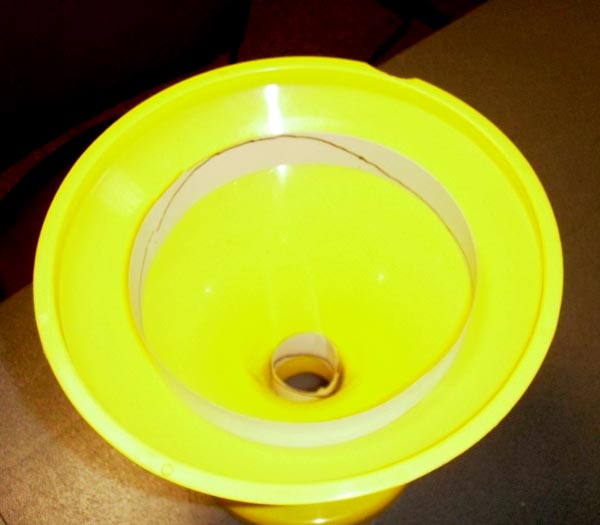Two sinewaves, one with a single wavelength, and another with two complete waves each with double the wavelength of the lowest energy state wavelength. This is a Vortx (sic) bank.

Orbitals

The Bohr model was replaced after a few years by Scrodinger's model which used 3 dimensional functions, the associated spherical legendre polynomials, which give the shapes of orbitals we have come to know and love.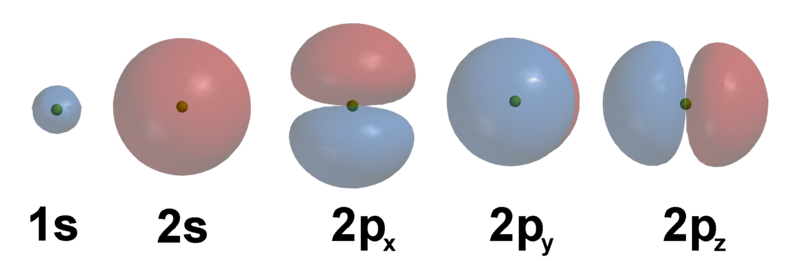These orbitals lead to energy levels of the atoms. When electrons change energy levels they emit or absorb photons. Usually 1 photon.

Energy Level Model, of a stool.

Computers can be used to display orbitals in 3D views.

Orbital Viewer PC or MAC http://www.orbitals.com/orb/index.html

Atom in a Box (MAC only) http://daugerresearch.com/orbitals/index.shtml

Fluorescence, Use energy levels to understand fluorescence.

Spectra,Examine the spectra of several light sources, energy levels help us understand spectra.

Floating Coins, a model of crystallization

 Scientific Explorations with Paul Doherty © 2009 17 November 2009